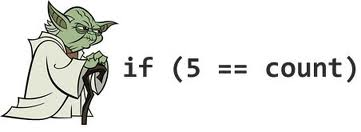# Never Greater

Quality
2021-07-13

Here is a quick tip to improve code readability. Never use the greater than or greater than or equal to operators.

Inverting terms so that they use a less than operator instead improves readability because the position of the variables are the same as their position as on a number line (or time line).

Look at these examples:

``` if ((x > lowerBound) && (x <= upperBound)) {} // e.g. 1a if ((x <= lowerBound) || (x > upperBound)) {} // e.g. 2a ```

Now rewrite the same only using less than signs.

``` if ((lowerBound < x) && (x <= upperBound)) {} // e.g. 1b if ((x <= lowerBound) || (upperBound < x)) {} // e.g. 2b ```

Logically examples 1a and 1b are the same, as are 2a and 2b. But in example 1b, it is clear `x` needs to be between the lower and upper bounds. It literal reads that way; that `x` is between the `lowerBound` and `upperBound` variables. In example 2b the code is testing that `x` is outside the lower and upper bounds.

It takes extra effort to read those 4 possible operators and try to visualize the proper order in your head. Writing the expressions out like a number line makes this much easier to visualize and allows the reader to spend their brainpower on more important things.

By extension, when writing SQL statements never use `Right Outer Join`. Always use `Left Outer Join` instead.

Some people write code like the first two examples (1a, 2a) because they are taught that the variable under test should always be on the left and the known value being tested against should always be on the left. To violate this rule is known as Yoda Conditions.

``` if (count == 5) {} // vs ```Yeah, that’s ugly. But is only applies to equality `==` and inequality `!=` operators. The rule about putting the variable under test on the left should not apply for the less than operator.

Happy coding.